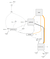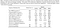Understanding Tensorflow Part 3

The term “Temporal Convolutional Networks” (TCNs) is a vague term that could represent a wide range of network architectures. In this post it is pointed specifically to one family of architectures proposed in the paper An Empirical Evaluation of Generic Convolutional and Recurrent Networks for Sequence Modeling:

Our aim is to distill the best practices in convolutional network design into a simple architecture.

The authors released the source code in PyTorch, which is well-written and easy to incorporate into your own projects. You can skip all the Tensorflow parts below and use their implementation instead if you just want to use TCNs with PyTorch.

In this post, we’ll learn how to write models with customized building blocks by implementing TCNs using tf.layers APIs.

20180528 Update (Gihub repo with links to all posts and notebooks):

Previous parts of this series:

An Overview of TCNs

The distinguishing characteristics of TCNs are: 1) the convolutions in the architecture are causal, meaning that there is no information “leakage” from future to past; 2) the architecture can take a sequence of any length and map it to an output sequence of the same length, just as with an RNN.

Dilated Causal Convolution

The most important component of TCNs is dilated causal convolution. “Causal” simply means a filter at time step t can only see inputs that are no later than t. Dilated convolution is well explained in this blog post. The point of using dilated convolution is to achieve larger receptive field with fewer parameters and fewer layers. (I also mentioned dilated causal convolution in the writeup of the Instacart competition).

Residual Blocks

A residual block stacks two dilated causal convolution layers together, and the results from the final convolution are added back to the inputs to obtain the outputs of the block. If the width(number of channels) of the inputs and the width(number of filters) of the second dilated causal convolution layers differs, we’ll have to apply an 1D convolution to the inputs before the adding the convolution outputs to match the widths.

Putting It Together

What TCNs do is simply stacking a number of residual blocks together to get the receptive field that we desire. If the receptive field is larger or equal to the maximum length of any sequences, the results of a TCN will be semantically equivalent to the results of a RNN.

Calculating Receptive Field

It’s important to know how to calculate the receptive field because you’ll need it to determine how many layers of residual blocks you need in the model.

Here we denote the number of previous time steps(history) the ith a dilated causal convolution layer can see as F(i).

For layer 0 (an imagined convolution as the initial case), F(0) = 1, as a causal convolution can always see its current time steps it’s at.

For layer 1, F(1) = F(0) + [kernel_size(n)-1] * dilation(n). It can see what the previous layer can see plus the position of the last kernel minus the position of the first. We can verify this using Figure 1 — F(1) = 1 + (3–1) * 1 = 3.

For layer 2, F(2) = F(1) + [kernel_size(n)-1] * dilation(n). Verify — F(2) = 3 + (3–1) * 1 = 5. This matches Figure 1c.

You should be able to see the pattern now. Generally, F(n) = F(n-1) + [kernel_size(n)-1] * dilation(n), where n means we’re at the nth dilated causal convolution layer since the input layer. Since every residual block has two identical dilated causal convolutions (same kernel sizes and dilations), we could simplifies the formula to F’(n) = F’(n-1) + 2 * [kernel_size(n)-1] * dilation(n), but n now means we are at the nth residual block.

If the kernel size is fixed, and the dilation of each residual block increases exponentially by 2, i.e. dilation(n) = 2^(n-1), we can expand the formula as F’(n) = 1 + 2 * (kernel_size-1) * (1 + 2 + 2² + … + 2^(n-1)) = 1 + 2*(kernel_size-1)*(2^n-1). Verify using Figure 1c — 1+2*(3–1)*(2¹-1)=5. You could verify the result with more residual blocks yourself.

So there it is, with a fixed kernel size and exponentially increasing dilations, TCN with n residual blocks will have a receptive field of
1 + 2*(kernel_size-1)*(2^n-1) at the final block. It most likely won’t match your maximum sequence length exactly, so you’ll have to decide to add one more block to make it larger than the maximum length, or sacrifice some of the older history.

Tensorflow Implementation with tf.layers

As before, the notebook with the source code use in the post is uploaded to Google Colab:

LINK TO THE NOTEBOOK

tf.layers

We’re going to use the tf.layers module to provide high-level abstraction for the implemented TCNs. The base layer class tf.layers.Layer is the foundation of all other layers in the module. The official documentation recommends descendants to this class implements the following three methods:

1. __init__() : Save configuration in member variables.
2. build() : Called once from __call__, when we know the shapes of inputs and dtype. Should have the calls to add_variable(), and then call the super's build().
3. call() :Called in __call__ after making sure build() has been called once. Should actually perform the logic of applying the layer to the input tensors (which should be passed in as the first argument).

(The descriptions above were directly copied from the documentation)

When in doubt, try to read the source code of a built-in layer and imitate what it does in those methods.

Dilated Causal Convolution

It’s quite simple to implement this since tf.layers.Conv1D already supports dilation through the dilation_rate parameter. What we need to do is to pad the start of the sequence with (kernel_size-1) * dilation zeros ourselves, and pass padding='valid'(basically means no padding) to the parent tf.layers.Conv1D. The padding will make the first output element only able to see the first input element (and the padding zeros).

Because of the restriction from other layers, CausalConv1D only support channels_last data format, i.e. input shape is always (batch_size, length, channels). It use tf.pad to pad the input tensor. Most of the lines are just capturing the initialization parameters of tf.layers.Conv1D.

Residual Blocks

Besides dilated causal convolution, we still need weight normalization, dropout, and the optional 1x1 Conv to complete the residual block.

I did not find an easy way to implement weight normalization in Tensorflow, so I replaced it with tf.contrib.layers.layernorm(layer normalization). They won’t be the same, but should have similar effects in stabilizing training. The layer normalization implementation basically assumes the channels are located at the last dimension of the input tensor, so the whole stack needs to use channels_last data format.

x = tf.contrib.layers.layer_norm(x) # using the shortcut

In the dropout section, we randomly drop out some of the channels across all time steps (a.k.a spatial dropout). tf.layers.Dropout layer has a parameter noise_shape that does exactly that. By setting the noise_shape to (batch_size, 1, channels), we select some channels for each example and set the dropout mask. Then the mask is broadcast to all time steps. (Check the notebook for a simple example.)

In the following implementation noise_shape is set to (1, 1, channels) to allow dynamic batch sizes. This will slow down convergence. If you want dynamic batch sizes with different masks for each example, you’ll have to override the _get_noise_shape() method to generate noise_shape dynamically.

self.dropout1 = tf.layers.Dropout(
self.dropout,
[tf.constant(1), tf.constant(1), tf.constant(self.n_outputs)])

Finally, the 1x1 convolution can easily be achieved with a tf.layers.Dense layer (it creates a projection at the last dimension):

if input_shape[channel_dim] != self.n_outputs:
self.down_sample = tf.layers.Dense(
self.n_outputs, activation=None)

The naming of the class follows the PyTorch implementation. Two dropout layers was created instead of one (same applies to layer normalization) simply to make Tensorboard create a cleaner graph visualization:

TCNs

All that is left to do is to stack residual blocks together and create dilations exponentially:

Note we can name each block manually with the name parameter, which will be shown in the Tensorboard:

TODO: Write Unit Tests

I haven’t figured out how to properly write unit tests against Tensorflow layers, but it should be a hard requirement if you want to use this implementation on real-world datasets.

Solve Sequential MNIST with TCN

Some differences comparing to the previous RNN models:

1. AdamOptimizer: Higher momentum usually works better with convolution neural networks than with RNNs.
2. No gradient clipping: Convolution neural networks does not has the problem of exploding gradients.
3. is_training placeholder: we need to disable dropout while predicting to have better predictions (otherwise you’ll have to do MC dropout). An example session run (in training): sess.run(train_op, feed_dict={X: batch_x, Y: batch_y, is_training: True})

We set kernel size to be 8 and number of stacked blocks to be 6, so the receptive field will be 1 + 2 * (8–1) * (2⁶-1) = 883, a bit larger than the maximum sequence length 784.Using TCNs to Solve (Permuted) Sequential MNIST

You can see in the notebook that a TCN with ~ 36K parameters converged faster and had better test accuracy than RNN from the previous notebook.Comparing TCN with three RNN variants. (Taken from the TCN paper)

Coming up: The Dataset API

We’ve been using the test set in the training process to pick the final model, which is a very bad practice. It makes the results from the two notebooks so far somewhat unreliable. In the next and probably the final part of this series, we’ll learn how to import the Fashion-MNIST dataset and create a proper validation set to evaluate our models.

The Artificial Impostor

Pretending to write about data science, deep learning, and some others (a.k.a. the whole AI package).

Welcome to a place where words matter. On Medium, smart voices and original ideas take center stage - with no ads in sight. Watch
Follow all the topics you care about, and we’ll deliver the best stories for you to your homepage and inbox. Explore
Get unlimited access to the best stories on Medium — and support writers while you’re at it. Just \$5/month. Upgrade